# Categorical Data

Categorical Data

Let us observe the following situation before starting:

The following pie chart depicts the results of a random poll on the choice of holiday locations of people in a city.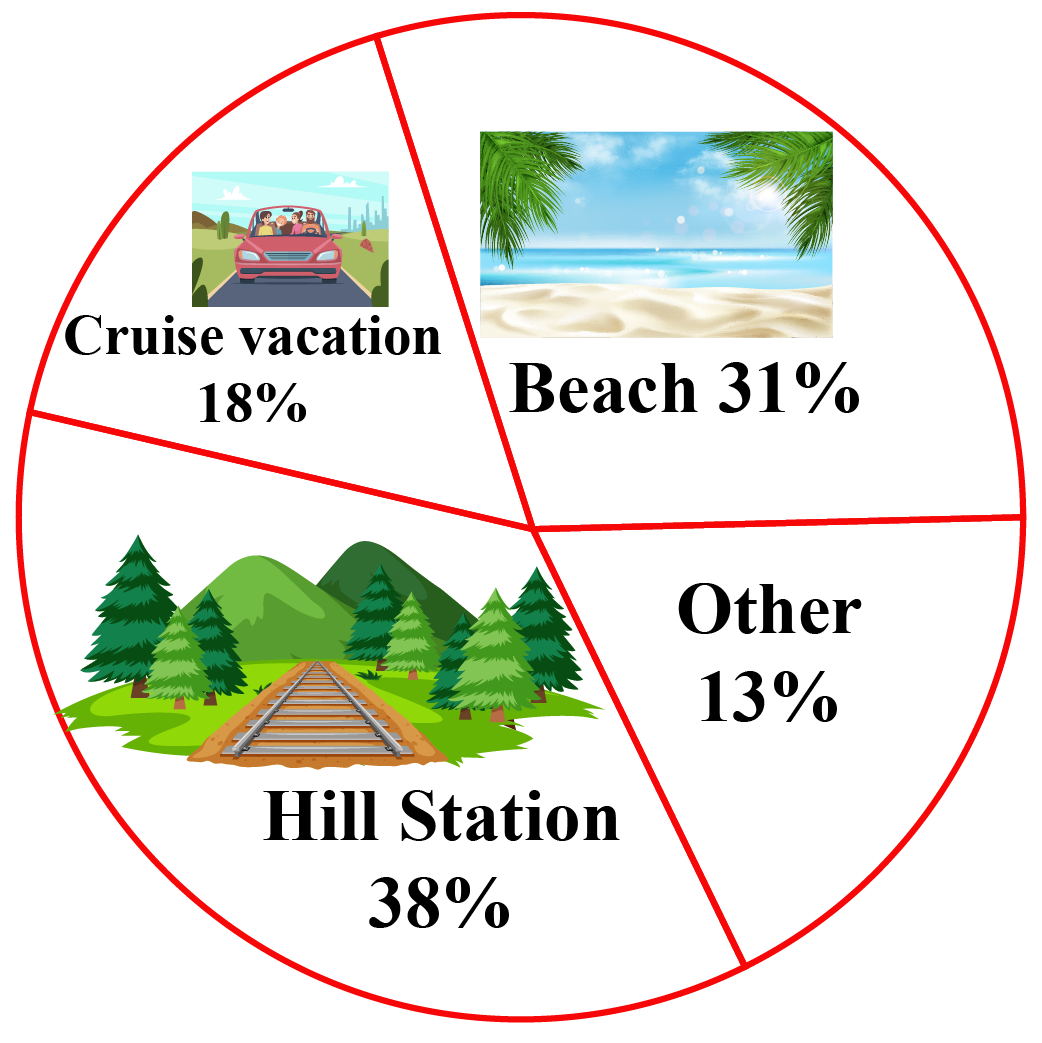Since the above recorded data includes the names of different types of locations under one category, will it be considered as categorical data?

It also has a percentage value of preferences for different locations which includes numerical data, so would it be considered as numerical data?

To find the answers to the above questions, check out this mini-lesson.

In this chapter, you will learn about categorical data examples, the distinction between categorical and numerical data, categorical data meaning, and the types of categorical data.

Check out the interactive examples and try your hand at solving a few practice questions at the end of the page.

## Lesson Plan

 1 What Is Categorical Data? 2 Important Notes on Categorical Data 3 Solved Examples on Categorical Data 4 Challenging Questions on Categorical Data 5 Interactive Questions on Categorical Data

## What Is Categorical Data?

Data is the term used to refer to a set of raw information gathered for a specific purpose.

Typically, data is divided into two categories. They are:

• Numerical Data (quantitative data)
• Categorical Data (qualitative data)

Categorical Data Meaning

Categorical data is the data grouped in the form of categories.

The grouping is done based on the qualitative aspects of the gathered raw information.

These qualitative aspects can include colors, age groups, food cuisines, sports, genders, shapes, etc.

This doesn't mean that categorical data has no relation with numerical values.

In some cases, categorical data might include numerical values to define the quantity of the grouped data.

Categorical Data Examples

The following images show how categorical data are grouped together.

Different seasons share a common category called "seasons."

Similarly, t-shirts of different sizes can be grouped together under the category "t-shirts."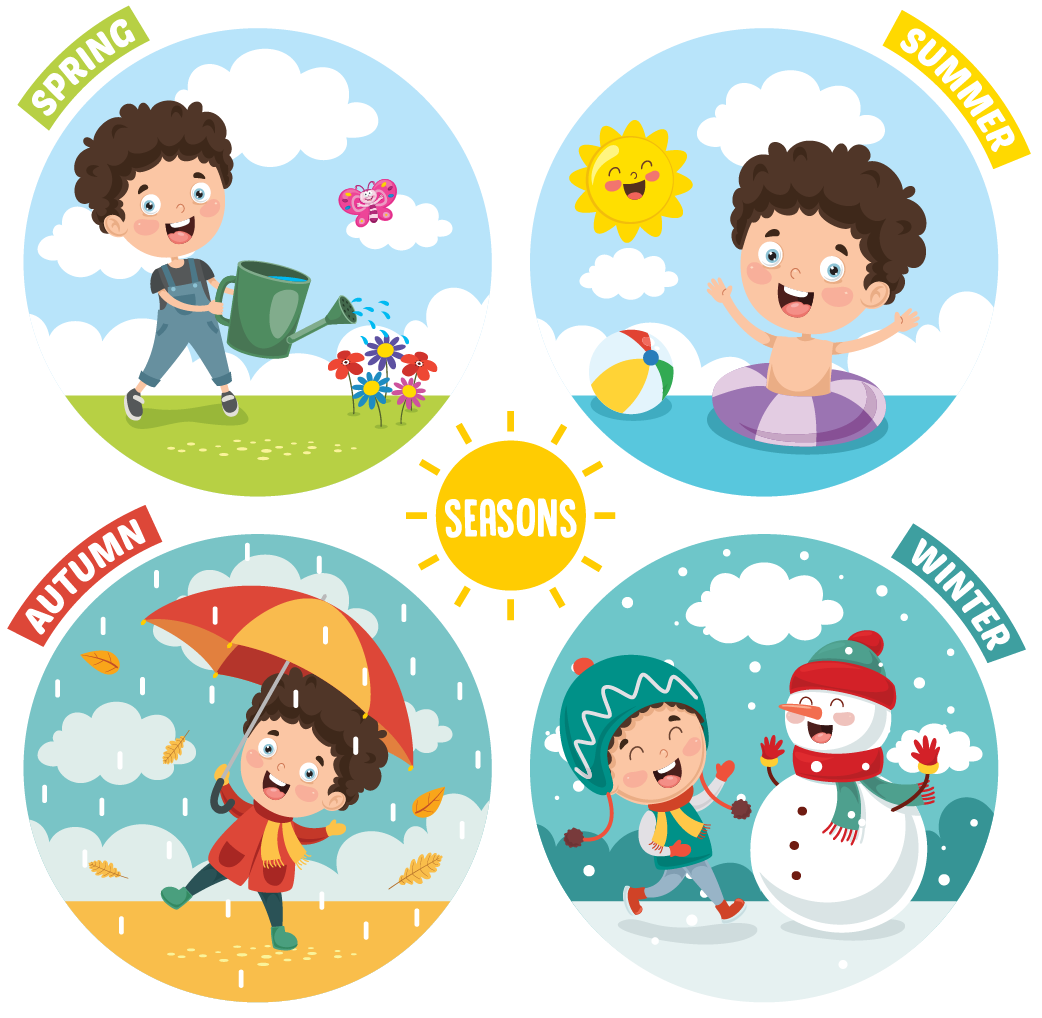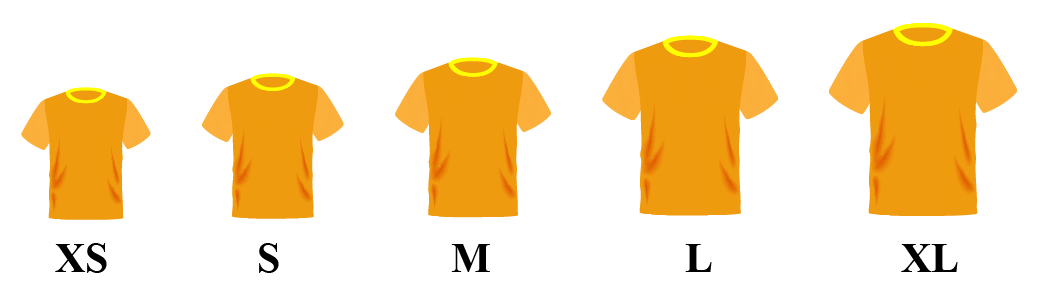The following table shows examples of categories and the objects under them.

Category Examples
Hair colorsPerfume brandsAge groupsFilm GenresFestivals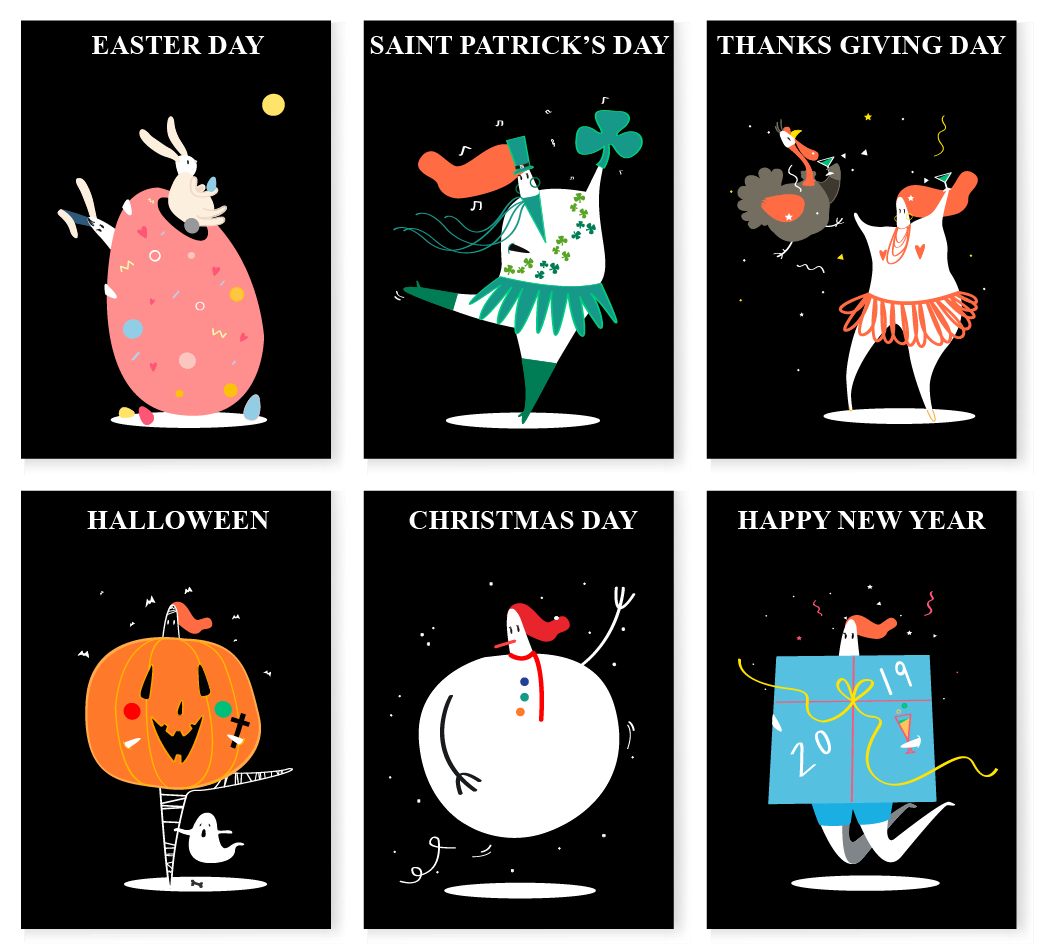## What Are the Types of Categorical Data?

There are two types of categorical data.

• Nominal categorical data
• Ordinal categorical data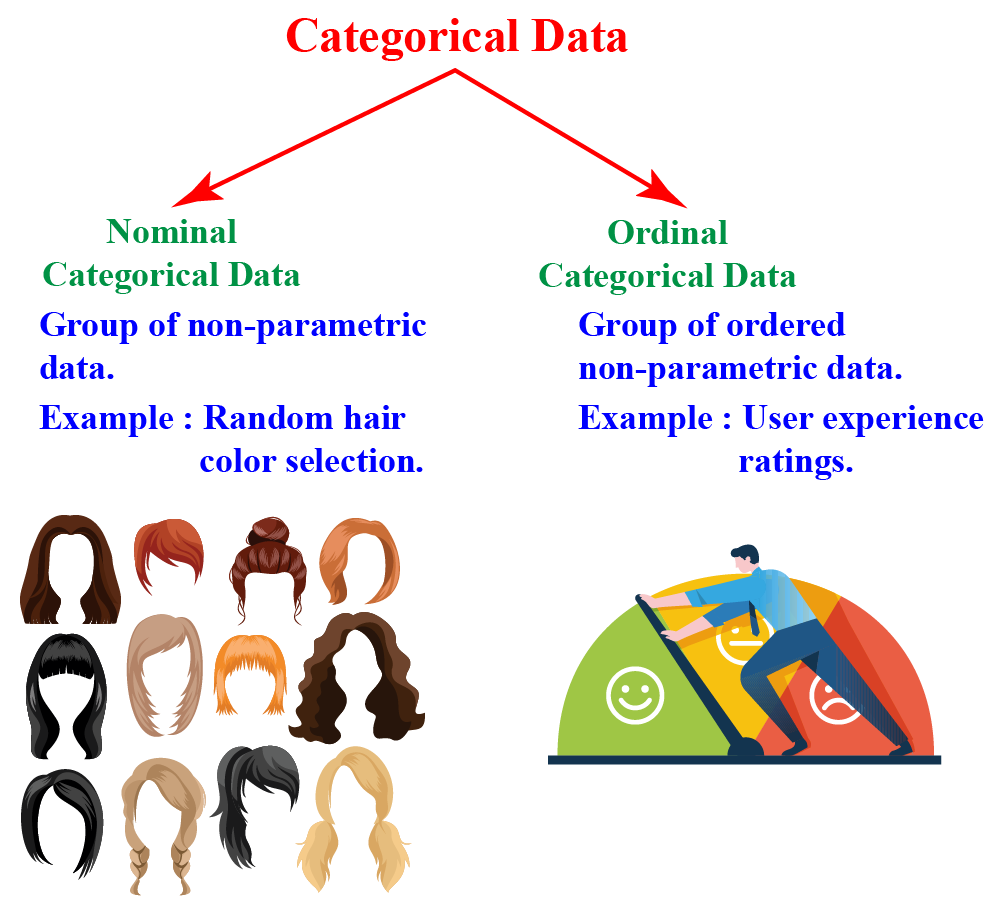Nominal categorical data

The word "Nominal" is derived from the Latin word "Nomen", which means name.

Thus, nominal categorical data includes "named" or "labeled" data, which does not take the numerical values of the data into consideration.

Examples include different writing genres like fiction and sci-fi, varieties of flowers, color hues, etc. are examples of nominal categorical data.

Ordinal categorical data

Ordinal categorical data has a certain "scale" or "measure" for the data grouped together.

The scale might not always be specific or standard.

This kind of data is generally ordered or measured.

Due to the presence of numerical values, this type of categorical data is said to exhibit the properties of both categorical data and numerical data.

They can be analyzed through grouping and they can be visually represented using bar graphs.

Examples include surveys that take numerical values into analysis for comparing grouped data under categorical variables.

The following pie chart depicts the results of a random poll on the choice of holiday locations of people in a city.## What Is a Categorical Variable?

A categorical variable is a variable that takes different values under different names or labels under grouped categorical data.

A categorical variable serves as the basic attribute of the data classified under a specific category.

Examples

Different shades of color, luxury brands, blood groups of a person, etc. are examples of categorical variables.

 Example 1

Anna is learning to classify objects under categorical data.

Can you help her name 3 categorical variables for the following object?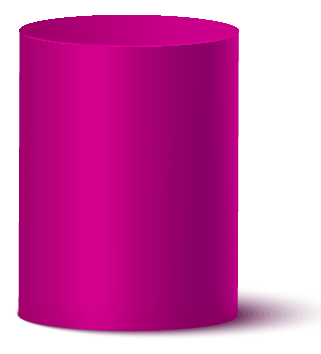Solution

Anna can categorize the above object under the following categorical variables:

• Cylinders
• Geometrical shapes
• Purple-colored objects
 Example 2

The following table depicts the percentage of people who prefer a certain movie genre.

Can you represent this categorical data using a pie chart?

Movie Genre Percentage of people
Comedy

20%

Romance

30%

Action

25%

Drama

5%

Sci-Fi

20%

Solution

The above grouped categorical data can be depicted using a pie chart as: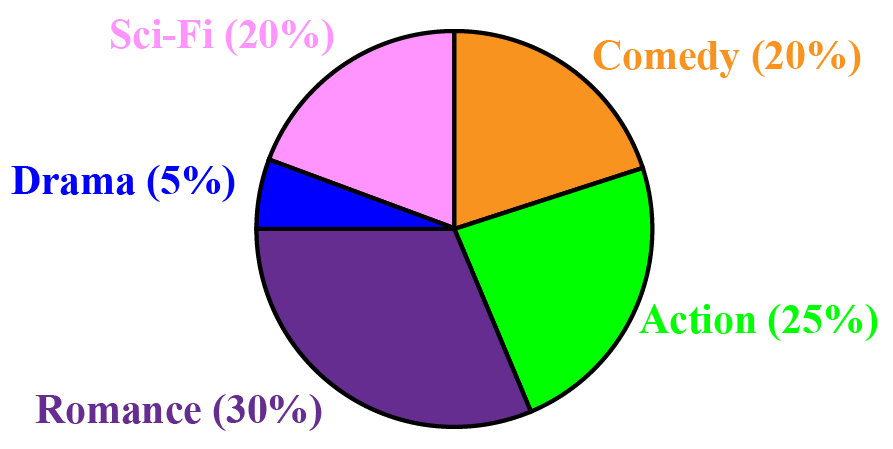Example 3

Can you help Amanda differentiate between nominal and ordinal categorical data?

Solution

The following table lists the differences between nominal and ordinal categorical data.

Nominal Categorical Data Ordinal Categorical Data
1. It is a group of non-parametric and non-ordered data.

1. It is a group of non-parametric ordered data.

2. The values are categorized as nominal based on their "names" or ''labels."

2. The values are categorized as ordinal on the basis of their numerical data.

3. Nominal categorical data is used to group similar objects under a similar category.

3. Ordinal categorical data is used to carry out analyses or studies on people's views or opinions.

4. Examples: Hair color, gender, country, race, etc.

4. Examples: The different positions secured by students in an examination, people's views on a survey, etc.
 Example 4

Ryan is studying how to sort nominal categories into desired groups.

Can you help him put the following colors into specific sections?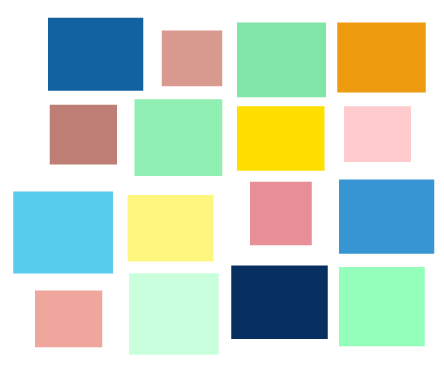Solution

The above colors can be arranged into nominal categorical data as shown below:Example 5

The following bar graph depicts the ordinal categorical data of the number of students whose birthdays fall on respective months.

Can you answer the following questions based on your observations of the graph?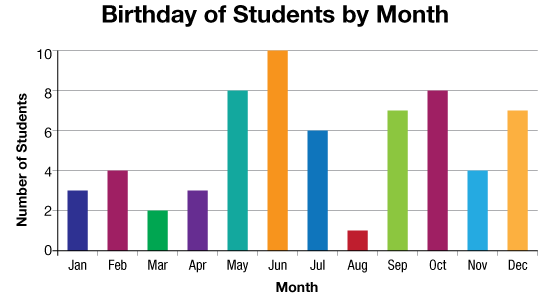• What is the total strength of students?
• Find the average of the three months with the most number of birthdays.
• Find the average of the four months with the least number of birthdays.

Solution

Based on the observations made from the bar graph:

Total number of students = 3 + 4 + 2 + 3 + 8 + 10 + 6 + 1 + 7 + 8 + 4 + 7 = 63 students

Average of the three months with the most number of birthdays = $$\frac{ 10 + 8 + 8 }{ 3 } = 8.667$$

Average of the four months with the least number of birthdays = $$\frac{1 + 2 + 3 + 3}{ 4} = 2.25$$Challenging Questions
• How would you differentiate numerical data from ordinal data?

## Interactive Questions

Here are a few activities for you to practice.

## Let's Summarize

The mini-lesson targeted the fascinating concept of categorical data. The math journey around categorical data starts with what a student already knows, and goes on to creatively crafting a fresh concept in the young minds. Done in a way that not only it is relatable and easy to grasp, but also will stay with them forever. Here lies the magic with Cuemath.

At Cuemath, our team of math experts is dedicated to making learning fun for our favorite readers, the students!

Through an interactive and engaging learning-teaching-learning approach, the teachers explore all angles of a topic.

Be it problems, online classes, doubt sessions, or any other form of relation, it’s the logical thinking and smart learning approach that we, at Cuemath, believe in.

## 1. Can number be categorical data?

Yes, numbers can be included as categorical data.

Numbers representing certain common labels and the numerical analysis of categorical data can be a part of grouping under categorical data.

## 2. Is a year considered as a categorical variable?

Yes, a year can be considered as a categorical variable.

Different months can be considered as categorical data.

## 3. Is age considered as nominal or ordinal categorical data?

Age can be categorized both as nominal and ordinal based on the usage.

Age, when used to depict certain order, comes under ordinal categorical data.

Nominal categorical data includes "named" or "labeled" data, which does not take the numerical values of the data into consideration.

Age as an aspect without comparison based on the order of numerical data comes under nominal categorical data.

More Important Topics
Numbers
Algebra
Geometry
Measurement
Money
Data
Trigonometry
Calculus
More Important Topics
Numbers
Algebra
Geometry
Measurement
Money
Data
Trigonometry
Calculus
Learn from the best math teachers and top your exams

• Live one on one classroom and doubt clearing
• Practice worksheets in and after class for conceptual clarity
• Personalized curriculum to keep up with school